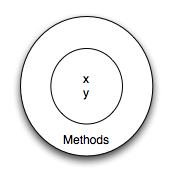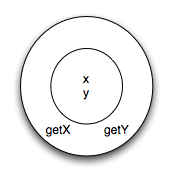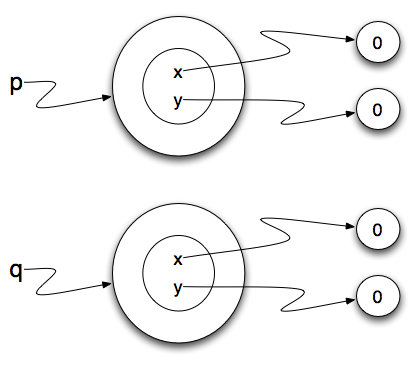# 20.3. User Defined Classes¶

We’ve already seen classes like `str`, `int`, `float` and `list`. These were defined by Python and made available for us to use. However, in many cases when we are solving problems we need to create data objects that are related to the problem we are trying to solve. We need to create our own classes.

As an example, consider the concept of a mathematical point. In two dimensions, a point is two numbers (coordinates) that are treated collectively as a single object. Points are often written in parentheses with a comma separating the coordinates. For example, `(0, 0)` represents the origin, and `(x, y)` represents the point `x` units to the right and `y` units up from the origin. This `(x,y)` is the state of the point.

Thinking about our diagram above, we could draw a `point` object as shown here.Some of the typical operations that one associates with points might be to ask the point for its x coordinate, `getX`, or to ask for its y coordinate, `getY`. You would want these types of functions available to prevent accidental changes to these instance variables since doing so would allow you to view the values without accessing them directly. You may also wish to calculate the distance of a point from the origin, or the distance of a point from another point, or find the midpoint between two points, or answer the question as to whether a point falls within a given rectangle or circle. We’ll shortly see how we can organize these together with the data.Now that we understand what a `point` object might look like, we can define a new class. We’ll want our points to each have an `x` and a `y` attribute, so our first class definition looks like this.

 ```1 2 3 4 5 6 7``` ```class Point: """ Point class for representing and manipulating x,y coordinates. """ def __init__(self): """ Create a new point at the origin """ self.x = 0 self.y = 0 ```

Class definitions can appear anywhere in a program, but they are usually near the beginning (after the `import` statements). The syntax rules for a class definition are the same as for other compound statements. There is a header which begins with the keyword, `class`, followed by the name of the class, and ending with a colon.

If the first line after the class header is a string, it becomes the docstring of the class, and will be recognized by various tools. (This is also the way docstrings work in functions.)

Every class should have a method with the special name `__init__`. This initializer method, often referred to as the constructor, is automatically called whenever a new instance of `Point` is created. It gives the programmer the opportunity to set up the attributes required within the new instance by giving them their initial state values. The `self` parameter (you could choose any other name, but nobody ever does!) is automatically set to reference the newly created object that needs to be initialized.

So let’s use our new Point class now. This next part should look a little familiar, if you remember some of the syntax for how we created instances of the Turtle class, in the chapter on Turtle graphics.

During the initialization of the objects, we created two attributes called x and y for each object, and gave them both the value 0. You will note that when you run the program, nothing happens. It turns out that this is not quite the case. In fact, two `Points` have been created, each having an x and y coordinate with value 0. However, because we have not asked the program to do anything with the points, we don’t see any other result.The following program adds a few print statements. You can see that the output suggests that each one is a `Point object`. However, notice that the `is` operator returns `False` meaning that they are different objects (we will have more to say about this in a later section).

A function like `Point` that creates a new object instance is called a constructor. Every class automatically uses the name of the class as the name of the constructor function. The definition of the constructor function is done when you write the `__init__` function (method) inside the class definition.

It may be helpful to think of a class as a factory for making objects. The class itself isn’t an instance of a point, but it contains the machinery to make point instances. Every time you call the constructor, you’re asking the factory to make you a new object. As the object comes off the production line, its initialization method is executed to get the object properly set up with it’s factory default settings.

The combined process of “make me a new object” and “get its settings initialized to the factory default settings” is called instantiation.

To get a clearer understanding of what happens when instantiating a new instance, examine the previous code using CodeLens.

Activity: CodeLens 20.3.3 (chp13_classes2a)

At Step 2 in the CodeLens execution, you can see that `Point` has been bound to an object representing the `Point` class, but there are not yet any instances. The execution of line 9, `p = Point()`, occurs at steps 3-5. First, at step 3, you can see that a blank instance of the class has been created, and is passed as the first (and only parameter) to the `__init__` method. That method’s code is executed, with the variable `self` bound to that instance. At steps 4 and 5, two instance variables are filled in: `x` and `y` are both set to `0`. Nothing is returned from the `__init__` method, but the point object itself is returned from the call to `Point()`. Thus, at step 7, `p` is bound to the new point that was created and initialized.

Skipping ahead, by the time we get to Step 14, `p` and `q` are each bound to different `Point` instances. Even though both have `x` and `y` instance variables set to `0`, they are different objects. Thus `p is q` evaluates to `False`.